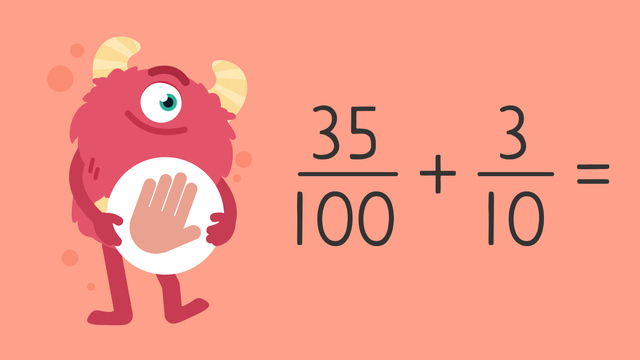# Adding Tenths and Hundredths — Let's Practice!Rating

Ø 5.0 / 1 ratings
The authorsTeam Digital
Adding Tenths and Hundredths — Let's Practice!
CCSS.MATH.CONTENT.4.NF.C.5

## Basics on the topicAdding Tenths and Hundredths — Let's Practice!

Today we are practicing adding tenths and hundredths with Razzi! This video contains examples to help you further practice and grow confident in this topic.

### TranscriptAdding Tenths and Hundredths — Let's Practice!

Razzi says get these item ready, because today we're going to practice adding tenths and hundredths. It's time to begin! Solve thirty-five hundredths plus three-tenths. Pause the video to work on the problem, and press play when you are ready to see the solution! We need like denominators to add. Start by converting three-tenths. Multiply the numerator and denominator by ten to get thirty-hundredths. Then, carry over one hundred for the denominator. Thirty-five plus thirty equals sixty-five. Did you also get sixty-five hundredths? Let's tackle the next problem! Solve three-hundredths plus five-tenths. Pause the video to work on the problem, and press play when you are ready to see the solution! We need like denominators to add. Start by converting five-tenths. Multiply the numerator and denominator by ten to get fifty-hundredths. Then, carry over one hundred for the denominator. Three plus fifty equals fifty-three. Did you also get fifty-three hundredths? Let's tackle the final problem! Solve thirty-hundredths plus two-tenths. This time, convert thirty-hundredths to tenths! Pause the video to work on the problem, and press play when you are ready to see the solution! Start by converting thirty hundredths to tenths. Divide the numerator and denominator by ten to get three-tenths. Then, carry over ten for the denominator. Three plus two equals five. Five tenths can be converted to one half! Did you also get five tenths or one half? Razzi had so much fun practicing with you today! See you next time!

## Adding Tenths and Hundredths — Let's Practice! exercise

Would you like to apply the knowledge you’ve learned? You can review and practice it with the tasks for the video Adding Tenths and Hundredths — Let's Practice!.
• ### Identify the expressions that are equivalent.

Hints

To compare the expressions, you must first create like-denominators.

The fractions, $\frac{55}{100}$ and $\frac{3}{100}$ share like denominators. The fractions, $\frac{55}{100}$ and $\frac{3}{10}$ do not.

Solution

When you add tenths and hundredths you need to first make sure the denominators are the same. By multiplying the numerator and the denominator of $\frac{3}{10}$ you make $\frac{30}{100}$, which you can then add to $\frac{35}{100}$. The same applies with $\frac{5}{10}$, $\frac{3}{10}$, and $\frac{4}{10}$.

• $\frac{3}{10}$ x 10 = $\frac{30}{100}$ = $\frac{35}{100}$ + $\frac{30}{100}$
• $\frac{5}{10}$ x 10 = $\frac{50}{100}$ = $\frac{3}{100}$ + $\frac{50}{100}$
• $\frac{6}{10}$ x 10 = $\frac{60}{100}$ = $\frac{60}{100}$ + $\frac{2}{100}$
• $\frac{4}{10}$ x 10 = $\frac{40}{100}$ = $\frac{40}{100}$ + $\frac{45}{100}$
• ### Identify the expressions that are equivalent.

Hints

To compare the expressions, you must first create like-denominators.

To compare the expressions, you must first create like-denominators.
The fractions, $\frac{55}{100}$ and $\frac{33}{100}$ share like denominators. The fractions, $\frac{55}{100}$ and $\frac{33}{10}$ do not.

When creating like denominators don't forget to divide or multiply the numerator as well!

Solution
• $\frac{50}{100}$ ÷ 10 = $\frac{5}{10}$
• $\frac{20}{100}$ ÷ 10 = $\frac{2}{10}$
• $\frac{40}{100}$ ÷ 10 = $\frac{4}{10}$
• $\frac{10}{100}$ ÷ 10 = $\frac{1}{10}$
• ### Solve the equations.

Hints

Use a pencil and paper to help you keep track of the steps.

With the equation, $\frac{35}{100}$ + $\frac{3}{10}$, and $\frac{3}{100}$ + $\frac{5}{10}$, it is easiest to multiply to create like denominators.

With the equation, $\frac{30}{100}$ + $\frac{2}{10}$, it is easiest to divide to create like denominators.

Do NOT simplify the fractions!

Solution
• $\frac{35}{100}$ + $\frac{3}{10}$ = $\frac{35}{100}$ + $\frac{30}{100}$ = $\frac{65}{100}$
• $\frac{3}{100}$ + $\frac{5}{10}$ = $\frac{3}{100}$ + $\frac{50}{100}$ = $\frac{53}{100}$
• $\frac{30}{100}$ + $\frac{2}{100}$ = $\frac{3}{10}$ + $\frac{2}{10}$ = $\frac{5}{10}$
• ### Simplify the solutions.

Hints

In the solution to $\frac{40}{100}$ + $\frac{40}{100}$, both 80 and 100 can be divided by 10. This solution can then be further simplified by dividing by 2.

Simplify the answer as many times as necessary.

Solution
• $\frac{40}{100}$ + $\frac{40}{100}$ = $\frac{80}{100}$
• $\frac{80}{100}$ ÷ $\frac{10}{10}$ = $\frac{8}{10}$
• $\frac{8}{10}$ ÷ $\frac{2}{2}$ = $\frac{4}{5}$
• ### Convert the tenths fraction to hundredths using multiplication.

Hints

Always remember to multiply both the numerator (above) and denominator (below) by the same number.

Multiply or divide to create like denominators before attempting to solve.

Solution
• 7 x 10 = 70, 10 x 10 = 100
• $\frac{70}{100}$ + $\frac{8}{100}$ = $\frac{78}{100}$
• ### Solve the equations.

Hints

In the equation, $\frac{20}{100}$ + $\frac{5}{10}$, $\frac{20}{100}$ can be divided by 10 to make equal denominators.

Remember to simplify as many times as necessary. If there is a common denominator between the numerator and denominator, divide again!

Solution
• $\frac{20}{100}$ + $\frac{5}{10}$ = $\frac{2}{10}$ + $\frac{5}{10}$ = $\frac{7}{10}$
• $\frac{30}{100}$ + $\frac{2}{10}$ = $\frac{3}{10}$ + $\frac{2}{10}$ = $\frac{5}{10}$ = $\frac{1}{2}$
• $\frac{3}{10}$ + $\frac{50}{100}$ = $\frac{3}{10}$ + $\frac{5}{10}$ = $\frac{8}{10}$ = $\frac{4}{5}$
• $\frac{4}{10}$ + $\frac{20}{100}$ = $\frac{4}{10}$ + $\frac{2}{10}$ = $\frac{6}{10}$ = $\frac{3}{5}$